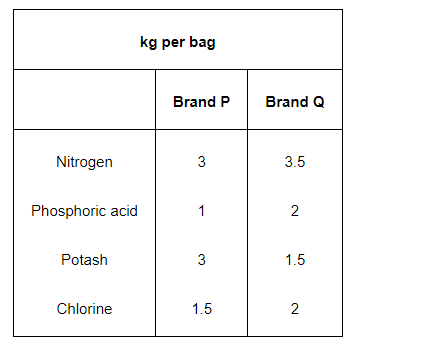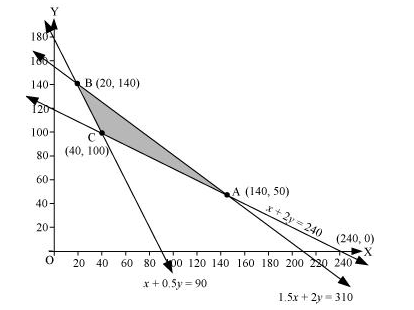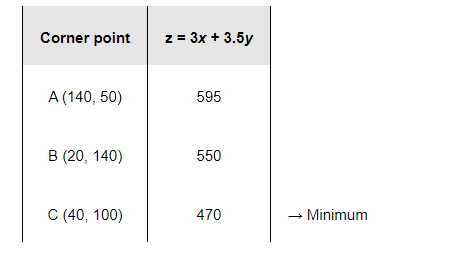# A fruit grower can use two types of fertilizer in his garden, brand P and brand Q.

Question:

A fruit grower can use two types of fertilizer in his garden, brand P and brand Q. The amounts (in kg) of nitrogen, phosphoric acid, potash, and chlorine in a bag of each brand are given in the table. Tests indicate that the garden needs at least 240 kg of phosphoric acid at least 270 kg of potash and at most 310 kg of chlorine.

If the grower wants to minimize the amount of nitrogen added to the garden, how many bags of each brand should be used? What is the minimum amount of nitrogen added in the garden?Solution:

Let the fruit grower use x bags of brand P and y bags of brand Q.

The problem can be formulated as follows.

Minimize $z=3 x+3.5 y$                            (1)

subject to the constraints,

$x+2 y \geq 240$                                 (2)

$x+0.5 y \geq 90$                                (3)

$1.5 x+2 y \leq 310$                            (4)

$x, y \geq 0$                                        (5)

The feasible region determined by the system of constraints is as follows.The corner points are A (240, 0), B (140, 50), and C (20, 140).

The values of z at these corner points are as follows.The maximum value of z is 470 at (40, 100).

Thus, 40 bags of brand P and 100 bags of brand Q should be added to the garden to minimize the amount of nitrogen.

The minimum amount of nitrogen added to the garden is 470 kg.